## Abstract

This article presents the MHD flow and heat transfer of a couple stress fluid over oscillatory stretching sheet embedded in a porous medium in the presence of heat source/sink. The unsteady flow problem is reduced to two coupled partial differential equations using dimensionless variables. Homotopy analysis method is employed to obtain the solution of these equations. Based on the solution of these equations an extensive analysis is performed to investigate the effects of various flow parameters on velocity and temperature fields, skin friction coefficient and Nusselt number. It is found that the presence of couple stress in viscous fluid increases the amplitude of oscillations in velocity and skin friction coefficient. It is also noticed that temperature increases by increasing heat source parameter. Moreover, the numerical values of local Nusselt number are calculated and shown in tabular form. It is found that the Nusselt number increases by increasing Prandtl number while it decreases by increasing couple stress parameter.

## Keywords

Boundary layer flow; Couple stress fluid; Oscillatory stretching sheet; Homotopy analysis method

## 1. Introduction

It is well established fact that Navier–Stokes equations are not adequate choice in describing the flows of non-Newtonian fluids. Non-Newtonian fluids frequently occur in many industrial and technological processes. If the flow of non-Newtonian fluid is considered over a stretching sheet then it become relevant to many industrial applications such a extrusion of plastic sheets, paper production, crystal growing and glass blowing, drawing of copper wires, continuous stretching of plastic films. The pioneering work on viscous flow due to continuous moving sheet was performed by Sakiadis . His analysis was extended for stretching sheet by Crane . Crane’s problem was extended by Rajagopal et al.  for viscoelastic fluid. Gupta and Gupta  extended the analysis of Crane by considering suction/blowing at the sheet surface. Dandapat and Gupta  discussed the heat transfer characteristics of viscoelastic fluid over a stretching sheet. A study of uniqueness of boundary value problem arising due to a stretching boundary in a viscous fluid was performed by McLeod and Rajagopal . Rollins and Vajravelu  discussed heat transfer characteristics of second-order fluid over a continuos stretching surface with internal heat generation or absorption. The stretching sheet problem for viscoelastic Oldroyd-B model was extended by Rajagopal and Bhatnagar . Cortell  presented a study of flow and heat transfer of hydromagnetic second-order fluid past a stretching sheet. In another paper , Cortell included magnetic and suction effects while discussing the flow and heat transfer of second grade fluid due to stretching sheet. The study of stagnation-point flow of micropolar fluid toward a stretching sheet was initiated by Nazar et al. . Combined forced and natural convection flow of micropolar fluid over a stretching sheet in the vicinity of stagnation point was discussed by Ishak et al. . Ariel  put forward a study of flow of second grade fluid past a radially stretching sheet. Ariel work was extended by Hayat and Sajid  to include the heat transfer characteristics. Some other contribution by Hayat and his co-workers regarding heat transfer analysis over a stretching surface can be found in references , ,  and . The investigation of non-similar flow of third order fluid over a stretching sheet was due to Sajid and Hayat . Hayat et al.  analyzed combined heat and mass transfer characteristics in boundary layer flow of viscoelastic fluid over linearly stretching vertical surface by using homotopy analysis method. Later on, Turkyilmazoglu  computed exact solution of hydromagnetic flow of viscoelastic fluid in the presence of Soret and Dufour effects. Turkyilmazoglu  also developed closed-form solutions for three dimensional flow and heat transfer of a electrically conducting viscoelastic fluid over a porous stretching/shrinking sheet. The multiplicity of the solutions in boundary layer flows over stretching/shrinking sheet is also addressed by Turkyilmazoglu. In fact, it was shown by him that multiple solutions exist for two-dimensional flow over stretching sheet and in the case of shrinking sheet triple solutions can be detected ,  and . In another paper, Turkyilmazoglu  computed exponential series type solution in the hydromagnetic and heat transfer in two–three dimensional flow over stretching or shrinking surface. According to him exponential series type solution exists with finite and infinite terms in the expansion depending upon prevailing parameters in the governing equations.

To best of our knowledge, Wang  initiated the study of viscous fluid due to oscillatory stretching sheet. His work was extended by Saddipa et al.  by using constitutive equation of Walter’s B model. Later on, Abbas et al.  complemented the Wang ’s analysis by including the effects of slip and heat transfer. They also utilized the constitutive relation of second order fluid  to study Wang’s problem. Zheng et al.  provided an analysis of unsteady heat and mass transfer in MHD flow over an oscillatory stretching sheet with Soret and Dufour effects. They have employed homotopy analysis method and delineated the effects of various emerging parameters on flow and heat transfer characteristics. Their study indicates a decrease in velocity of fluid by increasing unsteady parameter, magnetic parameter and suction/injection parameter. They also found an increase in thermal boundary layer thickness with magnetic parameter and unsteady parameter. Ali et al.  analyzed the unsteady flow of Jeffrey fluid over an oscillatory stretching surface. Khan et al.  used an implicit finite difference scheme to analyze the heat transfer effects in the hydromagnetic flow of viscoelastic fluid (second grade fluid) over an oscillatory stretching surface. However, so far no one has considered the study of unsteady boundary layer flow of a couple stress fluid through a porous medium over an oscillatory stretching surface in the presence of heat generation or absorption. Hence we feel appropriate to consider the study of such flows, as such type of flows may find a number of industrial applications. The theory of couple stress fluid was developed by Stokes  as a simple generalization of classical viscous theory that sustains couple stresses and body couples. The main effects of couple stress fluid are to introduce a size dependent effect which is not present in classical viscous theories. Blood, lubricants containing small amount of additives, electro-rheological and synthetic fluids are examples of couple stress fluid . Couple stress theory has been successfully applied to many problems in biomechanics and lubrications area by Srivastava , El-Shehawey and Mekheimer , Pal et al. , Chiang et al. , Naduvinamani et al. , Jian and Chen et al.  and Lu and Lin .

The presentation of the paper is as follows. Flow analysis is presented in Section 2. Homotopy analysis method is employed for solution of governing equations in Section 3. Convergence of solution is discussed in Section 4. Quantitative analysis of results is presented in Section 5. Final remarks are presented at the end of the paper in Section 6.

## 2. Flow analysis

Let us consider the unsteady two-dimensional magnetohydrodynamics flow of an incompressible fluid over an oscillatory stretching sheet. In a rectangular coordinate system ${\textstyle \left({\overline {x}}{\mbox{,}}{\overline {y}}\right)}$, the sheet is assumed to coincide with ${\textstyle {\overline {y}}=0}$ and flow takes place in the semi-infinite porous space ${\textstyle \left({\overline {y}}>0\right)}$ of constant permeability (see Fig. 1). A constant magnetic field of strength ${\textstyle B_{0}}$ is applied perpendicular to the stretching surface. It is assumed that the sheet is stretched with velocity ${\textstyle u_{\omega }=b{\overline {x}}sin\omega t}$ in which b   is the stretching rate and ${\textstyle \omega }$ denotes the angular velocity. Moreover, sheet is maintained at uniform temperature ${\textstyle T_{w}}$${\textstyle \left(>T_{\infty }\right)}$, where ${\textstyle T_{\infty }}$ is the temperature of ambient fluid. Now problem is to determine the velocity and temperature fields inside the fluid satisfying the boundary conditions at the wall and far away from the wall. To this end, we apply boundary layer approximation on continuity and momentum equations and write

 ${\frac {\partial u}{\partial {\overline {x}}}}+{\frac {\partial v}{\partial {\overline {y}}}}=$$0{\mbox{,}}$
(1)

 ${\frac {\partial u}{\partial t}}+u{\frac {\partial u}{\partial {\overline {x}}}}+$$v{\frac {\partial u}{\partial {\overline {y}}}}=\nu {\frac {{\partial }^{2}u}{\partial {\overline {y}}^{2}}}-$${\frac {{\eta }_{0}}{\rho }}\left[{\frac {{\partial }^{4}u}{\partial {\overline {y}}^{4}}}\right]-$${\frac {\sigma B_{0}^{2}}{\rho }}u-{\frac {\upsilon \phi }{k}}u{\mbox{,}}$
(2)

 ${\frac {\partial T}{\partial t}}+u{\frac {\partial T}{\partial {\overline {x}}}}+$$v{\frac {\partial T}{\partial {\overline {y}}}}={\frac {k}{\rho c_{p}}}{\frac {{\partial }^{2}T}{\partial {\overline {y}}^{2}}}+$${\frac {Q}{\rho c_{p}}}\left(T-T_{\infty }\right){\mbox{,}}$
(3)

where u and v   are velocity component along ${\textstyle {\overline {x}}}$ and ${\textstyle {\overline {y}}}$-directions, respectively, ${\textstyle \nu }$ is the kinematic viscosity, ${\textstyle \rho }$ is the density, ${\textstyle {\eta }_{0}}$ is the material constant for the couple stress fluid, Q   is the heat source coefficient, ${\textstyle \sigma }$ is electric conductivity and ${\textstyle B_{0}}$ is the strength of constant applied magnetic field. The flow is subjected to the following boundary conditions

 $u=u_{\omega }=b{\overline {x}}sin\omega t{\mbox{,}}\quad v=$$0{\mbox{,}}\quad {\frac {{\partial }^{2}u}{\partial {\overline {y}}^{2}}}=$$0{\mbox{,}}\quad T=T_{w}\quad at\quad {\overline {y}}=0{\mbox{,}}\quad t>0{\mbox{,}}$
(4)

 $u=0{\mbox{,}}\quad {\frac {\partial u}{\partial {\overline {y}}}}=$$0{\mbox{,}}\quad T\rightarrow T_{\infty }\quad \quad \quad at\quad \quad \quad {\overline {y}}\rightarrow \infty {\mbox{.}}$
(5)

The first two boundary conditions in (4) result from the assumption of no-slip at the surface while the third condition is consequence of the assumption of vanishing of couple stresses at the wall. Since flow is in unbounded domain that is why augmented boundary condition is imposed at ${\textstyle y\rightarrow \infty }$. To non-dimensionalize the flow problem, we use the dimensionless variables  and 

 $y={\sqrt {\frac {b}{\nu }}}{\overline {y}}{\mbox{,}}\quad \tau =$$t\omega {\mbox{,}}\quad u=b{\overline {x}}f_{y}\left(y{\mbox{,}}\tau \right){\mbox{,}}\quad v=$$-{\sqrt {\nu b}}f\left(y{\mbox{,}}\tau \right){\mbox{,}}$
(6)

 $\theta (y{\mbox{,}}\tau )={\frac {T-T_{\infty }}{T_{w}-T_{\infty }}}{\mbox{.}}$
(7)

In view of above transformations, the continuity equation is identically satisfied and Eqs. (2) and (3) are transformed as follows

 $f_{yyy}-{Sf}_{y\tau }-f_{y}^{2}+{ff}_{yy}-\beta f_{y}-{Kf}_{yyyyy}=$$0{\mbox{,}}$
(8)

 ${\theta }_{yy}+Pr\left(f{\theta }_{y}-S{\theta }_{\tau }+\right.$$\left.\lambda \theta \right)=0{\mbox{,}}$
(9)

where the subscript denotes differentiation with respect to y. The boundary conditions (4) and (5) take the following form

 $f_{y}\left(0{\mbox{,}}\tau \right)=sin\tau {\mbox{,}}\quad f\left(0{\mbox{,}}\tau \right)=$$0{\mbox{,}}\quad f_{yyy}\left(0{\mbox{,}}\tau \right)=$$0{\mbox{,}}\quad \theta (0{\mbox{,}}\tau )=1{\mbox{,}}$
(10)

 $f_{y}\left(\infty {\mbox{,}}\tau \right)=0{\mbox{,}}\quad f_{yy}\left(\infty {\mbox{,}}\tau \right)=$$0{\mbox{,}}\quad \theta (\infty {\mbox{,}}\tau )=0{\mbox{.}}$
(11)

In above equations ${\textstyle K={\eta }_{0}b/\rho {\upsilon }^{2}}$ is couple stress parameter, ${\textstyle S=\omega /b}$ is unsteady parameter, ${\textstyle \beta =\sigma B_{0}^{2}/\rho b+\upsilon \phi /kb}$ is the magneto-porous parameter, ${\textstyle Pr=\mu c_{p}/k}$ is the Prandtl number and ${\textstyle \lambda =Q/c\rho c_{p}}$ is the heat source ${\textstyle \left(\lambda >0\right)}$ or sink ${\textstyle \left(\lambda <0\right)}$ parameter. The parameter ${\textstyle K={\eta }_{0}\rho b/{\mu }^{2}=l^{2}b\rho /\mu }$ is called couple stress parameter. It has been pointed out by Stokes  that the effects of couple stress are quite large for large values of ${\textstyle K=l^{2}b\rho /\mu }$, where ${\textstyle b\rho /\mu }$ is a typical length scale associated with the flow and ${\textstyle l={\sqrt {\eta /\mu }}}$ is a material coefficient which is a function of molecular dimensions of the liquid. The parameter l will be greatly varied for different liquids. For instant, the length of polymer chain may be a million time the diameter of a water molecule. This is the size dependent effect associated with the couple stress fluid theory. For small value of K the effects of couple stress diminish and fluid behaves like a Newtonian fluid.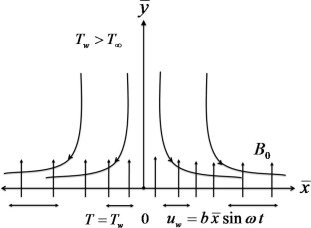Figure 1. Geometry of the problem.

The quantities of interest are skin friction coefficient and local Nusselt number which are defined as

 $C_{f}={\frac {{\tau }_{w}}{\rho u_{w}^{2}}}{\mbox{,}}\quad {Nu}_{x}=$${\frac {{\overline {x}}q_{w}}{k(T_{w}-T_{\infty })}}{\mbox{,}}$
(12)

where

 ${\tau }_{w}=\mu {\left({\frac {\partial u}{\partial {\overline {y}}}}\right)}_{{\overline {y}}=0}-$${\frac {{\eta }_{0}}{\rho }}{\left({\frac {{\partial }^{3}u}{\partial {\overline {y}}^{3}}}\right)}_{{\overline {y}}=0}{\mbox{,}}\quad \quad \quad \quad \quad q_{w}=$$-k{\left({\frac {\partial T}{\partial {\overline {y}}}}\right)}_{{\overline {y}}=0}{\mbox{,}}$
(13)

are skin friction and heat transfer at the wall, respectively. In view of transformations (6) and (7), we get

 ${Re}_{x}^{1/2}C_{f}=f_{yy}(0{\mbox{,}}\tau )-{Kf}_{yyyy}(0{\mbox{,}}\tau ){\mbox{,}}$
(14)
 ${Re}_{x}^{-1/2}{Nu}_{x}=-{\theta }_{y}(0{\mbox{,}}\tau ){\mbox{,}}$
(15)

where ${\textstyle {Re}_{x}=u_{w}{\overline {x}}/\nu }$ is the local Reynolds number.

## 3. Solution by homotopy analysis method

In many physical problems, the resulted differential equations are highly nonlinear nature. The computations of analytical or numerical solutions of such equations have set a challenge for the researchers. Homotopy analysis method (HAM) is one the best analytical methods to compute the series solution of such nonlinear partial as well as ordinary differential equations. This method tackles for strongly nonlinear problems even if a given nonlinear problem does not contain any small/large parameter. This method gives great freedom to choose and adjust the convergence region and rate of approximation. The homotopy analysis method is advantageous over routine numerical methods in the sense that it is free of rounding off errors which arise due to discretization procedure. Moreover, it does not require large computer memory and time . This method was successfully applied by researchers to solve various nonlinear problems in various disciplines of science and engineering , , , ,  and . It has been emphasized by Liao that the series solution obtained by HAM is purely analytic and he demonstrated this fact in number of articles for instance ,  and . Moreover, it has been proved by Turkyilmazoglu  that HAM can handle any type of nonlinearity by a suitable HAM formulation. For the series solution of Eqs. (8) and (9) subject to the boundary conditions (10) and (11), we employ homotopy analysis method (HAM). According to HAM the velocity and temperature fields can be represented as

 $f(y{\mbox{,}}\tau )=a_{0{\mbox{,}}0}^{0}+\sum _{n=0}^{\infty }\sum _{k=0}^{\infty }\sum _{j=0}^{\infty }a_{n{\mbox{,}}k}^{j}y^{k}sin(j\tau )exp(-$$ny){\mbox{,}}$
(16)
 $\theta (y{\mbox{,}}\tau )=b_{0{\mbox{,}}0}^{0}+\sum _{n=0}^{\infty }\sum _{k=0}^{\infty }\sum _{j=0}^{\infty }b_{n{\mbox{,}}k}^{j}y^{k}sin(j\tau )exp(-$$ny){\mbox{,}}$
(17)

where ${\textstyle a_{n{\mbox{,}}k}^{j}}$ and ${\textstyle b_{n{\mbox{,}}k}^{j}}$ are the coefficients to be determined. The appropriate initial guesses for ${\textstyle f(y{\mbox{,}}\tau )}$ and ${\textstyle \theta (y)}$ are

 $f_{0}(y{\mbox{,}}\tau )={\frac {1}{2}}sin\tau (3-3exp(-y)-$$yexp(-y)){\mbox{,}}$
(18)
 ${\theta }_{0}(y)=exp(-y){\mbox{.}}$
(19)

Further we choose

 ${\mbox{£}}_{f}(f)={\frac {{\partial }^{3}f}{\partial y^{3}}}-$${\frac {\partial f}{\partial y}}{\mbox{,}}$
 ${\mbox{£}}_{\theta }(f)={\frac {{\partial }^{2}f}{\partial y^{2}}}-$$f{\mbox{,}}$

as auxiliary linear operators having following properties

 ${\mbox{£}}_{f}[C_{1}+C_{2}exp(-y)+C_{3}exp(y)]=0{\mbox{,}}$
(20)
 ${\mbox{£}}_{\theta }[C_{4}exp(y)+C_{5}exp(-y)]=0{\mbox{,}}$
(21)

where ${\textstyle C_{i}}$${\textstyle \left(i=1-5\right)}$ are arbitrary constants. If ${\textstyle h_{f}}$ and ${\textstyle h_{\theta }}$ denotes the nonzero auxiliary parameters then zeroth order deformation problems are constructed as follows:

 $(1-p){\mbox{£}}_{f}[{\overset {\mbox{ˆ}}{f}}(y{\mbox{,}}\tau {\mbox{;}}p)-$$f_{0}(y{\mbox{,}}\tau )={ph}_{f}N_{f}[{\overset {\mbox{ˆ}}{f}}(y{\mbox{,}}\tau {\mbox{;}}p)]{\mbox{,}}$
(22)

 $(1-p){\mbox{£}}_{f}[{\overset {\mbox{ˆ}}{\theta }}(y{\mbox{,}}\tau {\mbox{;}}p)-$${\theta }_{0}(y{\mbox{,}}\tau )={ph}_{\theta }N_{\theta }[{\overset {\mbox{ˆ}}{\theta }}(y{\mbox{,}}\tau {\mbox{;}}p){\mbox{,}}{\overset {\mbox{ˆ}}{f}}(y{\mbox{,}}\tau {\mbox{;}}p)]{\mbox{,}}$
(23)
 ${\overset {\mbox{ˆ}}{f}}(0{\mbox{,}}\tau {\mbox{;}}p)=0{\mbox{,}}{\frac {\partial {\overset {\mbox{ˆ}}{f}}(y{\mbox{,}}\tau {\mbox{;}}p)}{\partial y}}=$$sin\tau {\mbox{,}}\quad {\frac {\partial {\overset {\mbox{ˆ}}{f}}(y{\mbox{,}}\tau {\mbox{;}}p)}{\partial y}}=$$0{\mbox{,}}\quad {\frac {{\partial }^{2}{\overset {\mbox{ˆ}}{f}}(y{\mbox{,}}\tau {\mbox{;}}p)}{\partial y^{2}}}=$$0{\mbox{,}}$
(24)
 ${\overset {\mbox{ˆ}}{\theta }}(0{\mbox{,}}\tau {\mbox{;}}p)=$$1{\mbox{,}}\quad {\overset {\mbox{ˆ}}{\theta }}(\infty {\mbox{,}}\tau {\mbox{;}}p)=$$0{\mbox{,}}$

where ${\textstyle p\in [0{\mbox{,}}1]}$ is an embedding parameter and nonlinear operators ${\textstyle N_{f}}$ and ${\textstyle N_{\theta }}$ are

 $N_{f}[{\overset {\mbox{ˆ}}{f}}(y{\mbox{,}}\tau {\mbox{;}}p)]=$$-K{\frac {{\partial }^{5}{\overset {\mbox{ˆ}}{f}}(y{\mbox{,}}\tau {\mbox{;}}p)}{\partial y^{5}}}+$${\frac {{\partial }^{3}{\overset {\mbox{ˆ}}{f}}(y{\mbox{,}}\tau {\mbox{;}}p)}{\partial y^{3}}}-$$S{\frac {{\partial }^{2}{\overset {\mbox{ˆ}}{f}}(y{\mbox{,}}\tau {\mbox{;}}p)}{\partial y\partial \tau }}+$${\overset {\mbox{ˆ}}{f}}(y{\mbox{,}}\tau {\mbox{;}}p){\frac {{\partial }^{2}{\overset {\mbox{ˆ}}{f}}(y{\mbox{,}}\tau {\mbox{;}}p)}{\partial y^{2}}}-$${\left({\frac {\partial {\overset {\mbox{ˆ}}{f}}(y{\mbox{,}}\tau {\mbox{;}}p)}{\partial y}}\right)}^{2}-$$\beta {\frac {\partial {\overset {\mbox{ˆ}}{f}}(y{\mbox{,}}\tau {\mbox{;}}p)}{\partial y}}{\mbox{,}}$
(25)
 $N_{\theta }[{\overset {\mbox{ˆ}}{\theta }}(y{\mbox{,}}\tau {\mbox{;}}p){\mbox{,}}{\overset {\mbox{ˆ}}{f}}(y{\mbox{,}}\tau {\mbox{;}}p)]=$${\frac {{\partial }^{2}{\overset {\mbox{ˆ}}{\theta }}(y{\mbox{,}}\tau {\mbox{;}}p)}{\partial y^{2}}}+$$Pr\left({\overset {\mbox{ˆ}}{f}}(y{\mbox{,}}\tau {\mbox{;}}p){\frac {\partial {\overset {\mbox{ˆ}}{\theta }}(y{\mbox{,}}\tau {\mbox{;}}p)}{\partial y}}-\right.$$\left.S{\frac {\partial {\overset {\mbox{ˆ}}{\theta }}(y{\mbox{,}}\tau {\mbox{;}}p)}{\partial \tau }}+\right.$$\left.\lambda {\overset {\mbox{ˆ}}{\theta }}(y{\mbox{,}}\tau {\mbox{;}}p)\right){\mbox{.}}$
(26)

The zeroth-order deformation problems defined above have the following solutions corresponding to ${\textstyle p=0}$ and ${\textstyle p=1}$

 ${\overset {\mbox{ˆ}}{f}}(y{\mbox{,}}\tau {\mbox{;}}0)=f_{0}(y{\mbox{,}}\tau ){\mbox{,}}\quad {\overset {\mbox{ˆ}}{f}}(y{\mbox{,}}\tau {\mbox{;}}1)=$$f(y{\mbox{,}}\tau ){\mbox{,}}$
(27)
 ${\overset {\mbox{ˆ}}{\theta }}(y{\mbox{,}}\tau {\mbox{;}}0)=$${\theta }_{0}(y{\mbox{,}}\tau ){\mbox{,}}\quad {\overset {\mbox{ˆ}}{\theta }}(y{\mbox{,}}\tau {\mbox{;}}1)=$$\theta (y{\mbox{,}}\tau ){\mbox{.}}$
(28)

Expanding ${\textstyle {\overset {\mbox{ˆ}}{f}}(y{\mbox{,}}\tau {\mbox{;}}p)}$ and ${\textstyle {\overset {\mbox{ˆ}}{\theta }}(y{\mbox{,}}\tau {\mbox{;}}p)}$ in a Taylor’s series with respect to p, we get

 ${\overset {\mbox{ˆ}}{f}}(y{\mbox{,}}\tau {\mbox{;}}p)={\overset {\mbox{ˆ}}{f}}_{0}(y{\mbox{,}}\tau )+$$\sum _{m=1}^{\infty }f_{m}(y{\mbox{,}}\tau )p^{m}{\mbox{,}}$
(29)
 ${\overset {\mbox{ˆ}}{\theta }}(y{\mbox{,}}\tau {\mbox{;}}p)=$${\overset {\mbox{ˆ}}{\theta }}_{0}(y{\mbox{,}}\tau )+\sum _{m=1}^{\infty }{\theta }_{m}(y{\mbox{,}}\tau )p^{m}{\mbox{,}}$
(30)
 $f_{m}(y{\mbox{,}}\tau )={\frac {1}{m!}}{\frac {{\partial }^{m}{\overset {\mbox{ˆ}}{f}}(y{\mbox{,}}\tau {\mbox{;}}p)}{\partial p^{m}}}{\mbox{,}}\quad {\theta }_{m}(y{\mbox{,}}\tau )=$${\frac {1}{m!}}{\frac {{\partial }^{m}{\overset {\mbox{ˆ}}{\theta }}(y{\mbox{,}}\tau {\mbox{;}}p)}{\partial p^{m}}}{\mbox{,}}$
(31)

Differentiating zeroth order deformations equations (22) and (23)m-times with respect to p  , then setting ${\textstyle p=0}$ and finally dividing by ${\textstyle m!}$, we get the following mth-order deformation problem

 ${\mbox{£}}_{f}[{\overset {\mbox{ˆ}}{f}}_{m}(y{\mbox{,}}\tau )-$${\varkappa }_{m}f_{m-1}(y{\mbox{,}}\tau )=h_{f}R_{m}^{f}(y{\mbox{,}}\tau ){\mbox{,}}$
(32)
 ${\mbox{£}}_{f}[{\overset {\mbox{ˆ}}{\theta }}_{m}(y{\mbox{,}}\tau )-$${\varkappa }_{m}{\theta }_{m-1}(y{\mbox{,}}\tau )=h_{\theta }R_{m}^{\theta }(y{\mbox{,}}\tau ){\mbox{,}}$
(33)
 $f_{m}(0{\mbox{,}}\tau {\mbox{;}}p)=0{\mbox{,}}{\frac {\partial f_{m}(y{\mbox{,}}\tau {\mbox{;}}0)}{\partial y}}_{y=0}=$${\frac {\partial f_{m}(y{\mbox{,}}\tau {\mbox{;}}0)}{\partial y}}_{y=\infty }=$${\frac {\partial f_{m}^{2}(y{\mbox{,}}\tau {\mbox{;}}0)}{\partial y^{2}}}_{y=\infty }=$$0{\mbox{,}}$
(34)
 ${\theta }_{m}(0)=0{\mbox{,}}{\theta }_{m}(\infty )=0{\mbox{,}}$
(35)
 $R_{m}^{f}(y{\mbox{,}}\tau )=-K{\frac {{\partial }^{5}f_{m-1}}{\partial y^{5}}}+$${\frac {{\partial }^{3}f_{m-1}}{\partial y^{3}}}-S{\frac {{\partial }^{2}f_{m-1}}{\partial y\partial \tau }}-$$\beta {\frac {\partial f_{m-1}}{\partial y}}+\sum _{k=0}^{m-1}\left(f_{m-1-k}{\frac {{\partial }^{2}f_{k}}{\partial y^{2}}}-\right.$$\left.{\frac {f_{m-k-1}}{\partial y}}{\frac {\partial f_{k}}{\partial y}}\right){\mbox{,}}$
(36)
 $R_{m}^{\theta }(y{\mbox{,}}\tau )={\frac {{\partial }^{2}{\theta }_{m-1}}{\partial y^{2}}}-$$Pr\left[S{\frac {\partial {\theta }_{m-1}}{\partial \tau }}\right]+$$Pr\left(\sum _{k=0}^{m-1}\left[f_{k}{\frac {\partial {\theta }_{m-1-k}}{\partial y}}\right]+\right.$$\left.\lambda {\theta }_{m-1}\right){\mbox{,}}$
(37)
 ${\varkappa }_{m}={\begin{array}{ll}0{\mbox{,}}&m\leqslant 1{\mbox{,}}\\1{\mbox{,}}&m>1{\mbox{,}}\end{array}}$
(38)

## 4. Convergence of HAM solution

It is well established fact that the convergence of the HAM solution is largely dependent on the proper choice of the auxiliary parameters ${\textstyle h_{f}}$ and ${\textstyle h_{\theta }}$. For a particular set of parameters the convergence region can be obtained by plotting h-curves. Figure 2 and Figure 3 present two such curves showing the plausible values of ${\textstyle h_{f}}$ and ${\textstyle h_{\theta }}$ for a given set of parameters. We note from these figures that for convergent solution ${\textstyle -1.2\leqslant h_{f}<-0.2}$ and ${\textstyle -1.5\leqslant h_{\theta }<-0.2}$. The purpose of showing such curves is just to emphasize that in principle for any physical choice of parameters of the problem, a convergent solution can be obtained. The values of ${\textstyle f^{''}(0{\mbox{,}}\tau )}$ obtained by HAM in the present paper are also compared with the corresponding values reported in Refs. , ,  and  in Table 1 and Table 2. Both tables show that our results are in excellent agreement with the existing results in the literature.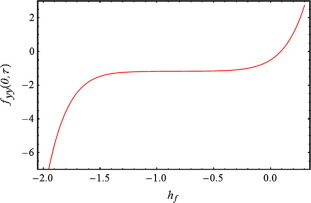Figure 2. The h  -curve for velocity at 7th order of approximation: with ${\textstyle K=0.1{\mbox{,}}\beta =0.2{\mbox{,}}S=0.1}$ and ${\textstyle \tau =0.5\pi }$.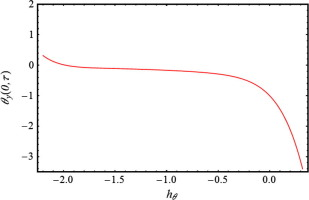Figure 3. The h  -curve for temperature at 7th order of approximation with ${\textstyle K=0.1{\mbox{,}}S=0.1{\mbox{,}}\beta =0.2{\mbox{,}}\lambda =}$$0.5{\mbox{,}}Pr=0.1$ and ${\textstyle \tau =0.5\pi }$.

Table 1. Comparison of values of ${\textstyle f^{''}(0{\mbox{,}}\tau )}$ with Refs.  with ${\textstyle K=0}$ and .
S ${\textstyle \beta }$ ${\textstyle \tau }$ Zheng et al.  Abbas et al.  Present results
${\textstyle 1.0}$ 12 ${\textstyle 1.5\pi }$ ${\textstyle 11.678565}$ ${\textstyle 11.678656}$ ${\textstyle 11.678565}$
${\textstyle 5.5\pi }$ ${\textstyle 11.678706}$ ${\textstyle 11.678707}$ ${\textstyle 11.678706}$
${\textstyle 9.5\pi }$ ${\textstyle 11.678656}$ ${\textstyle 11.678656}$ ${\textstyle 11.678656}$

Table 2. Comparison of values of ${\textstyle f^{''}(0{\mbox{,}}\tau )}$ for different values of ${\textstyle \beta }$ when ${\textstyle K=0{\mbox{,}}S=0}$ and ${\textstyle \tau =\pi /2}$.
${\textstyle \beta }$ Hayat et al.  Turkyilmazoglu  Present results
0 ${\textstyle -1.000000}$ ${\textstyle -1.00000000}$ ${\textstyle -1.000000}$
${\textstyle 0.5}$ ${\textstyle -1.224747}$ ${\textstyle -1.22474487}$ ${\textstyle -1.224747}$
${\textstyle 1.0}$ ${\textstyle -1.414217}$ ${\textstyle -1.41421356}$ ${\textstyle -1.414217}$
${\textstyle 1.5}$ ${\textstyle -1.581147}$ ${\textstyle -1.58113883}$ ${\textstyle -1.581147}$
${\textstyle 2.0}$ ${\textstyle -1.732057}$ ${\textstyle -1.73205081}$ ${\textstyle -1.732057}$

## 5. Results and discussion

The homotopy analysis method is a powerfull technique to solve nonlinear partial and ordinary differential equations with initial and boundary conditions. The set of nonlinear partial differential equations (8) and (9) with boundary conditions (10) and (11) is solved analytically by means of homotopy analysis method and the effects of involved parameters are illustrated through graphs. In this section, we are going to present the detail analysis of flow and heat transfer of couple stress fluid over oscillating stretching surface in the presence of heat source and sink.

Fig. 4(a) and (b) are plotted to show the behavior of couple stress parameter K   and magneto-porous parameter ${\textstyle \beta }$ on the time series of velocity ${\textstyle f^{'}}$ at a fixed location ${\textstyle y=0.25}$ from the sheet, respectively. In Fig. 4(a) the effects of couple stress parameter K   are illustrated by keeping ${\textstyle S=1}$ and ${\textstyle \beta =0.1}$. From this figure we observe that an increase in couple stress parameter K results in an increase in amplitude of velocity. This is perhaps due to the presence of couple stresses. Fig. 4(b) points out the effects of magneto-porous parameter ${\textstyle \beta }$ by keeping other parameters constant. This figure shows that the amplitude of velocity decreases by increasing magneto-porous parameter β.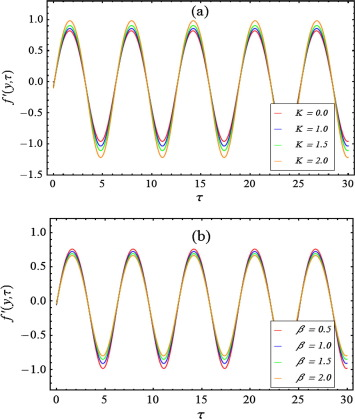Figure 4. Time series of the velocity profile ${\textstyle f^{'}}$ at a distance ${\textstyle y=0.25}$ from the sheet in the time period ${\textstyle \tau \in \left[0{\mbox{,}}10\pi \right]}$: (a) The effects of couple stress parameter K   with ${\textstyle S=1}$ and ${\textstyle \beta =0.1}$, (b) The effects of magneto-porous parameter ${\textstyle \beta }$ with ${\textstyle S=0.5}$ and ${\textstyle K=0.1}$.

Fig. 5(a)–(d) demonstrate the effects of couple stress parameter K   on velocity profile ${\textstyle f^{'}}$ at four different time instants ${\textstyle \tau =8.5\pi {\mbox{,}}\tau =9\pi {\mbox{,}}\tau =9.5\pi }$ and ${\textstyle \tau =10\pi }$. Fig. 5(a) characterizes the effects of couple stress parameter K   on the velocity profile ${\textstyle f^{'}}$ at ${\textstyle \tau =8.5\pi }$. This figure depicts that the velocity ${\textstyle f^{'}}$ decrease as we increase K. This decrease in velocity results in decrease in boundary layer thickness. In Fig. 5(b), the behavior of couple stress parameter K   on the ${\textstyle f^{'}}$ is shown at ${\textstyle \tau =9\pi }$. This figure shows an increase in magnitude of velocity at this instant. The effects of K   at ${\textstyle \tau =9.5\pi }$ and ${\textstyle \tau =10\pi }$ are illustrated in Fig. 5(c) and (d), respectively. It is observed from these figures that at ${\textstyle \tau =9.5\pi }$ and ${\textstyle \tau =10\pi }$, the velocity ${\textstyle f^{'}}$ decreases with K  . It is interesting to note that the effects of couple stress are to the increase the amplitude of velocity when ${\textstyle f^{'}=1}$ at the surface. For other instants when ${\textstyle f^{'}=-1}$ or 0, the magnitude of velocity decreases due to the presence of couple stresses.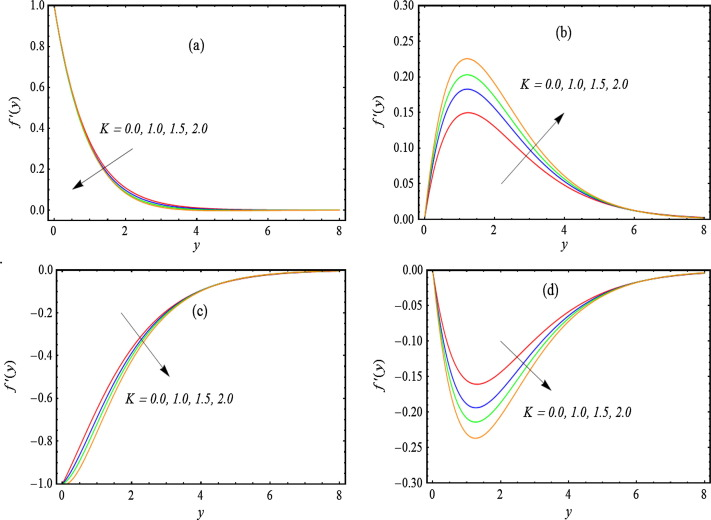Figure 5. Transverse profiles of the velocity field ${\textstyle f^{'}}$ for four different values of couple stress parameter K   in the fifth period ${\textstyle \tau \in \left[8\pi {\mbox{,}}10\pi \right]}$ for which a periodic velocity field has been reached: (a) ${\textstyle \tau =8.5\pi }$, (b) ${\textstyle \tau =9\pi }$, (c) ${\textstyle \tau =9.5\pi }$ and (d) ${\textstyle \tau =10\pi }$, with ${\textstyle S=1}$ and ${\textstyle \beta =0.1}$.

The effects of magneto-porous parameter ${\textstyle \beta }$ on velocity are illustrated in Fig. 6(a)–(d) at four different times keeping other parameters fixed. In Fig. 6(a) the effects of magneto-porous parameter ${\textstyle \beta }$ are plotted at ${\textstyle \tau =8.5\pi }$. From this figure it can be examined that velocity decreases with increase in magneto-porous parameter ${\textstyle \beta }$. The effects of magneto-porous parameter on velocity at other three time instants are illustrated in Fig. 6(c) and (d). Here, again it is observed that the magnitude of velocity decreases by increasing ${\textstyle \beta }$.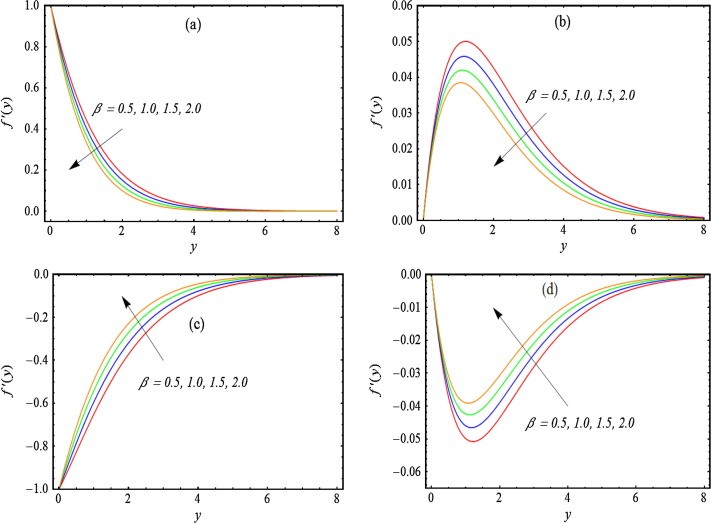Figure 6. Transverse profiles of the velocity field ${\textstyle f^{'}}$ for four different values of magneto-porous parameter ${\textstyle \beta }$ in the fifth period ${\textstyle \tau \in \left[8\pi {\mbox{,}}10\pi \right]}$ for which a periodic velocity field has been reached: (a) ${\textstyle \tau =8.5\pi }$, (b) ${\textstyle \tau =9\pi }$, (c) ${\textstyle \tau =9.5\pi }$, and (d) ${\textstyle \tau =10\pi }$, with ${\textstyle S=0.5}$ and ${\textstyle K=0.1}$.

The variation of skin friction coefficient with couple stress parameter K   and magneto-porous parameter ${\textstyle \beta }$ in the first five periods ${\textstyle \tau \in [0{\mbox{,}}10\pi ]}$ is shown in Fig. 7(a) and (b), respectively. Fig. 7(a) shows the time series of skin friction coefficient for different values of couple stress parameter K   by taking ${\textstyle S=0.1}$ and ${\textstyle \beta =0.1}$. From this figure we observe that skin friction coefficient varies periodically due to periodic motion of the surface and its amplitude increases with couple stress parameter K  . This observation reflects that skin friction for a Newtonian fluid flowing over an oscillatory stretching sheet is less in comparison with its value for a couple stress fluid performing the same motion. The effects of magneto-porous parameter ${\textstyle \beta }$ on skin friction coefficient are illustrated in Fig. 7(b). This figure depicts that amplitude of skin friction coefficient increases by increasing magneto-porous parameter ${\textstyle \beta }$. Such effects of ${\textstyle \beta }$ and M are expected because the fact that magnetic force and drag force offered by the porous medium acts as a resistance to the flow.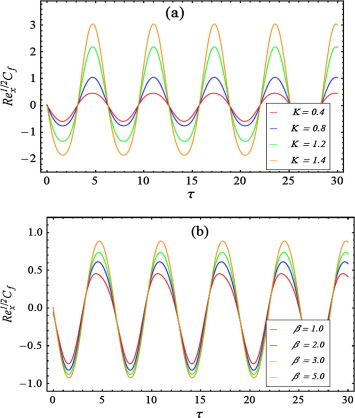Figure 7. Time series of the skin-friction coefficient ${\textstyle {Re}_{x}^{1/2}C_{f}}$ in the first five periods ${\textstyle \tau \in [0{\mbox{,}}10\pi ]}$: (a) effects of couple stress parameter K   with ${\textstyle S=0.1}$ and ${\textstyle \beta =0.1}$ and (b) effects of magneto-porous parameter ${\textstyle \beta }$ with ${\textstyle S=0.5}$ and ${\textstyle K=0.1}$.

Figure 8, Figure 9, Figure 10, Figure 11 and Figure 12 present the effects of Prandtl number Pr  , magneto-porous parameter ${\textstyle \beta }$, couple stress parameter K   and heat source/sink parameter ${\textstyle \lambda }$ on the temperature field. Fig. 8 reveals the effects of Prandtl number Pr on temperature profile for both heat source and sink cases. It is seen that temperature is a decreasing function of Pr in both cases. The fact is that the increase in Prandtl number results in low thermal conductivity, as a result conduction as well as thermal boundary layer thickness decreases and hence we observe a decrease in temperature. It is observed that the presence of heat sink enhances the decrease in temperature by increasing Prandtl number. In Fig. 9, the influence of magneto-porous parameter ${\textstyle \beta }$ on temperature field is explained graphically by keeping other parameters fixed. This figure shows that temperature increases with magneto-porous parameter ${\textstyle \beta }$. The influence of couple stress parameter K on temperature is shown in Fig. 10, which depicts that increase in couple stress parameter results in increase in temperature. Since we are discussing the temperature field in the presence of heat source/sink so effects of this parameter are very important for both the cases. The effects of heat source/sink parameter are illustrated in Figure 11 and Figure 12 by keeping ${\textstyle Pr=0.4{\mbox{,}}\beta =0.5{\mbox{,}}K=0.1{\mbox{,}}S=0.1}$. It is noted from Fig. 11 that as we increase the strength of the heat source, the temperature increases. This is due to the fact that heat source can add more heat to the stretching sheet which increases its temperature and thus the temperature of fluid rises. Moreover, the thermal boundary layer is also found to increase by increasing the strength of heat source. Fig. 12 is plotted to observe the effects of heat sink by keeping other parameters constant. This figure shows opposite results i.e., the temperature decreases by increasing the strength of heat sink. This is because, when strength of heat sink increases, more heat is removed from the sheet and thus a decrease in thermal boundary-layer thickness and temperature is observed. This result is of key importance for the flows where heat transfer is of prime importance. The numerical values of Nusselt number are shown in Table 3. It is found that the Nusselt number increases with Prandtl number while it decreases with increase in heat source, couple stress and magneto-porous parameter.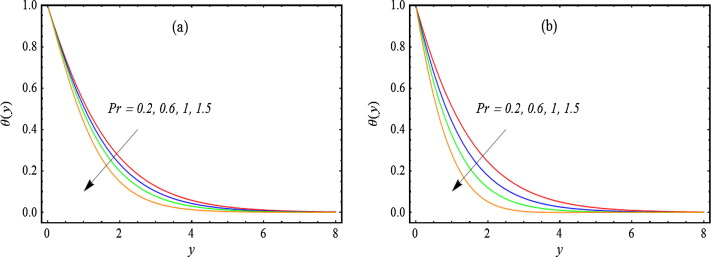Figure 8. The effects of Prandtl number Pr   on temperature field ${\textstyle \theta }$ at ${\textstyle \tau =8.5\pi }$ with ${\textstyle K=0.1{\mbox{,}}S=0.1{\mbox{,}}\beta =0.2}$, (a) in heat source case ${\textstyle \lambda =0.3}$, (b) in heat sink case ${\textstyle \lambda =-0.3}$.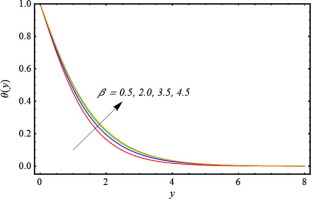Figure 9. The effects of magneto-porous parameter ${\textstyle \beta }$ on temperature field ${\textstyle \theta }$ at ${\textstyle \tau =8.5\pi }$ with ${\textstyle Pr=1.5{\mbox{,}}K=0.1{\mbox{,}}S=0.1{\mbox{,}}\lambda =0.3}$.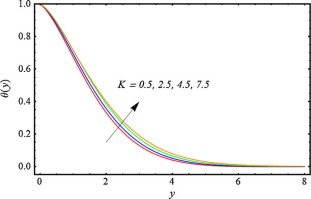Figure 10. The effects of couple stress number K   on temperature field ${\textstyle \theta }$ at ${\textstyle \tau =8.5\pi }$ with ${\textstyle Pr=1.5{\mbox{,}}\beta =5{\mbox{,}}\lambda =0.4{\mbox{,}}S=}$$0.6$.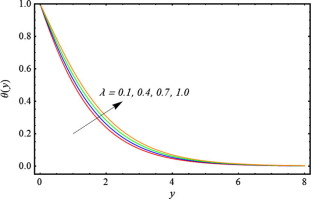Figure 11. The effects of heat source parameter ${\textstyle \lambda }$ on temperature field ${\textstyle \theta }$ at ${\textstyle \tau =8.5\pi }$ with ${\textstyle Pr=0.4{\mbox{,}}\beta =0.5{\mbox{,}}K=0.1{\mbox{,}}S=0.1}$.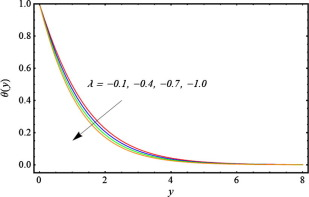Figure 12. The effects of heat sink parameter ${\textstyle \lambda }$ on temperature field ${\textstyle \theta }$ at ${\textstyle \tau =8.5\pi }$ with ${\textstyle Pr=0.4{\mbox{,}}\beta =0.5{\mbox{,}}K=0.1{\mbox{,}}S=0.1}$.

Table 3. The numerical values of the Local Nusselt number for different values of Prandtl number Pr, couple stress parameter K  , magneto-porous parameter ${\textstyle \beta }$ and heat source parameter ${\textstyle \lambda }$ at time ${\textstyle \tau =\pi /2}$ with ${\textstyle S=1}$.
Pr ${\textstyle \lambda }$ K ${\textstyle \beta }$ ${\textstyle {Re}_{x}^{-1/2}{Nu}_{x}}$
${\textstyle 0.5}$ ${\textstyle 0.2}$ ${\textstyle 5.0}$ ${\textstyle 5.0}$ ${\textstyle 0.596791}$
${\textstyle 1.0}$ ${\textstyle 0.634885}$
${\textstyle 1.5}$ ${\textstyle 0.671806}$
${\textstyle 2.0}$ ${\textstyle 0.705075}$
${\textstyle 0.5}$ ${\textstyle 0.2}$ ${\textstyle 0.596791}$
${\textstyle 0.4}$ ${\textstyle 0.547204}$
${\textstyle 0.6}$ ${\textstyle 0.496478}$
${\textstyle 0.8}$ ${\textstyle 0.44459}$
${\textstyle 0.2}$ ${\textstyle 0.2}$ ${\textstyle 0.573683}$
${\textstyle 0.4}$ ${\textstyle 0.573021}$
${\textstyle 0.8}$ ${\textstyle 0.572122}$
${\textstyle 1.0}$ ${\textstyle 0.571885}$
${\textstyle 5.0}$ ${\textstyle 0.5}$ ${\textstyle 0.643221}$
${\textstyle 1.5}$ ${\textstyle 0.630873}$
${\textstyle 2.5}$ ${\textstyle 0.619684}$
${\textstyle 3.5}$ ${\textstyle 0.609657}$

## 6. Concluding remarks

Flow and heat transfer of a couple stress fluid over an oscillatory stretching sheet in the presence of heat source/sink is investigated using HAM. It is observed that flow and heat transfer characteristics are greatly influenced by the presence of couple stress and heat source/sink parameter. In fact, the skin friction coefficient increases by increasing couple stress and magneto-porous parameter. It is also interesting to note that rate of heat transfer is enhanced by increasing magneto-porous parameter, heat source and couple stress parameter, while it decreases for large values of Prandtl number and heat sink parameter. Such observations may have interesting implications where it is desired to reduce skin friction and enhance heat transfer.

## Acknowledgments

We are grateful to the reviewers for their useful comments. The second author is grateful to the Higher Education Commission of Pakistan for financial assistance.

### Document informationPublished on 12/04/17

Licence: Other

### Document Score0

Views 94
Recommendations 0